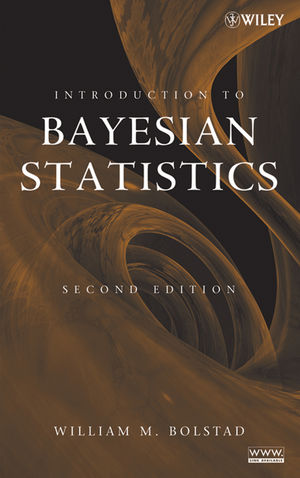understanding computational Bayesian statistics

(This post has been contributed by Christian Robert.)

I have just finished reading this book by Bill Bolstad (University of Waikato, New Zealand) which a previous ‘Og post pointed out when it appeared, shortly after our Introducing Monte Carlo Methods with R. My family commented that the cover was nicer than those of my books, which is true. Before I launch into a review, let me warn the ‘Og reader that, as an author of three books on computational Bayesian statistics, I cannot be very objective on the topic: I do favour the way we approached Bayesian computational methods and, after reading Bolstad’s Understanding computational Bayesian statistics, would still have written the books the way we did. Be warned, thus.

Understanding computational Bayesian statistics is covering the basics of Monte Carlo and (fixed dimension) Markov Chain Monte Carlo methods, with a fair chunk dedicated to prerequisites in Bayesian statistics and Markov chain theory. Even though I have only glanced at the table of contents of Bolstad’s Introduction to Bayesian Statistics [using almost the same nice whirl picture albeit inbronze rather than cobalt], it seems to me that the current book is the continuation of the earlier one, going beyond the Binomial, Poisson, and normal cases, to cover generalised linear models, via MCMC methods. (In this respect, it corresponds to Chapter 4 of Bayesian Core.) The book is associated with Minitab macros and an R package (written by James Curran), Bolstad2, in continuation of Bolstad, written for Introduction to Bayesian Statistics. Overall, the level of the book is such that it should be accessible to undergraduate students, MCMC methods being reduced to Gibbs, random walk and independent Metropolis-Hastings algorithms, and convergence assessments being done via autocorrelation graphs, the Gelman and Rubin (1992) intra-/inter-variance criterion, and a forward coupling device. The illustrative chapters cover logistic regression (Chap. 8), Poisson regression (Chap. 9), and normal hierarchical models (Chap. 10). Again, the overall feeling is that the book should be understandable to undergraduate students, even though it may make MCMC seem easier than it is by sticking to fairly regular models. In a sense, it is more a book of the [roaring MCMC] 90’s in that it does not incorporate advances from 2000 onwards (as seen from the reference list) like adaptive MCMC and the resurgence of importance sampling via particle systems and sequential Monte Carlo.

0 Responses to “understanding computational Bayesian statistics”